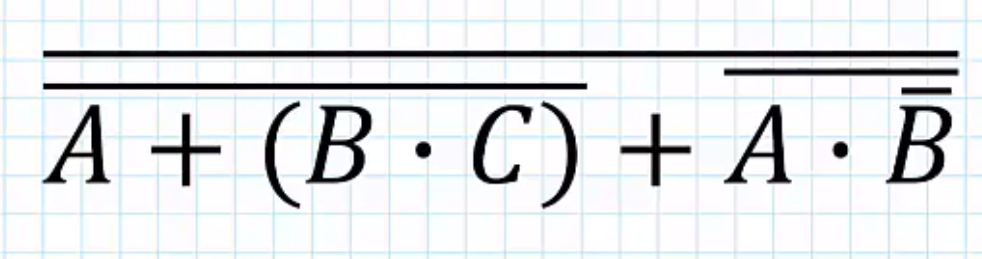## Forum Posts

tylerdee7
Sep 09, 2021
In ACSL Contest Prep
Today's class was about computer number systems: binary, octal, and hexadecimal. Addition and subtraction methods were also discussed. Homework: 1. (The numbers in this equation are all binary) 10101 + 1000110 + 111 = ? 2. (The numbers in this equation are all binary) 10001 - 111 = ? 3. (The numbers in this equation are all octal) 7 + 7 - 3 = ?
0
7
67
tylerdee7
Jun 24, 2021
In CS Fundamentals for ACSL
Convert the following into both prefix and postfix: 1+(5/2*2^2)-3 (9+1)/2*(3+7) Bonus Question: Let A be a variable for a number. Given the following postfix equation: 2A*1-7A*+5+ = 49 What value of A makes this equation true?
0
1
27
tylerdee7
Jul 23, 2020
In CS Fundamentals for ACSL
The following list is a Stack. Solve the following problem: AABBCDA Pop() Pop() Push(A) Push(E) Pop() Push(D) Pop() Push(A)
0
9
28
tylerdee7
Jul 11, 2020
In CS Fundamentals for ACSL
Simplify the following boolean algebra expression:0
10
62
tylerdee7
Jul 09, 2020
In CS Fundamentals for ACSL
Find f(18,6) given:0
6
42
T

Forum Moderator
More actions